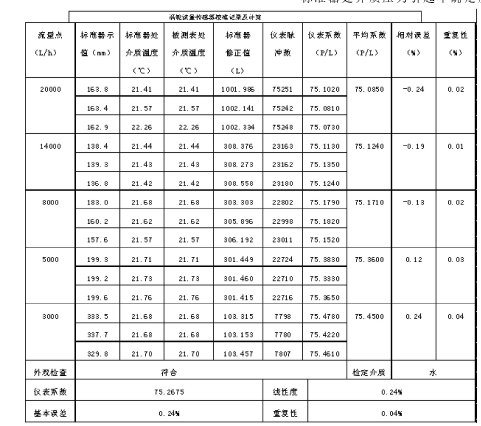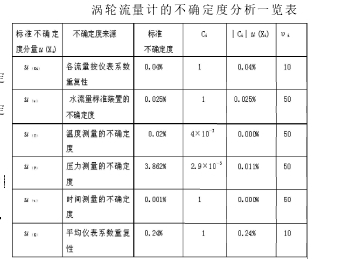0517-86996066
18915186518

#### 联系我们0517-86996066

JJG198-1994《速度式流量计》检定规程；测量环境：温度(5～35)℃；相对湿度（45～85）%RH；测量标准：用静态容积法流量标准装置作为测量标准；测量方法：采用比较测量法进行检定；测量对象：选取上海虹益的LWGY-40涡轮流量计进行评定。

（一）流量计算公式当工作流体为液体时：QV=V20[1+βθ（θ-20）]QV——累积体积流量V20——在20℃的工作量器的容积βθ——工作量器材料的体膨胀系数θ——试验时工作量器中的液体温度（二）流量计仪表系数K第i个流量点第j次检定，流量计仪表系数Kij为pijKij=Nij/Qvij｛1＋β［（ts）ij－tij］｝｛1－κ［（ps）ij－tij］｝Nij——流量计在一段时间内的累积脉冲数Qvij——标准器在相同时间内给出的体积值。主要由随机效应引入的不确定度分量和由系统效应引入的不确定度分量。以涡轮流量计为被测量对象，用水流量标准装置静态容积法进行检定，检定数据如下表（K）=0.04%  灵敏系数C1=1（二）水流量标准装置的不确定度（v） 检定涡轮流量计用流量标准装置的不确定为0.05%,k=2（v）=0.05%/2=0.025%灵敏系数C2=1（三）温度测量的不确定度（T）采用允许误差为±0.5℃的温度传感器（T1）=0.5/3000=0.017%按矩形分布考虑（T2）=U（T1）/=0.01%标准器处介质温度引起不确定度的灵敏系数为C31=4×10被测表处介质温度引起不确定度的灵敏系数为C31=－4×10（T）=2×0.01%×4×10=8×10（四）压力测量的不确定度（P）所用压力计满量程为600KPa,允许误差为满量程的1.6%，

（P1）=（600×1.6%）/287=3.345% 按矩形分布考虑（P2）=（P1）/=1.931%标准器处介质压力引起不确定度的灵（五）时间测量的不确定度（t）计时器的允许误差为0.001s,一次检定***短时间为60s（t1）=0.001/60=0.0017% 按矩形分布考虑（t）=0.0017%/=0.001%灵敏系数C5=1（六）平均仪表系数重复性（K）（K）=0.24%   灵敏系数C6=1涡轮流量计的不确定度分析一览表（一）各流量点仪表系数重复性（Ki）将各流量点仪表系数重复性（Ki）的***大值代入（K）=0.04%  灵敏系数C1=1（二）水流量标准装置的不确定度（v） 检定涡轮流量计用流量标准装置的不确定为0.05%,k=2（v）=0.05%/2=0.025%灵敏系数C2=1（三）温度测量的不确定度（T）采用允许误差为±0.5℃的温度传感器（T1）=0.5/3000=0.017%按矩形分布考虑（T2）=U（T1）/=0.01%标准器处介质温度引起不确定度的灵敏系数为C31=4×10被测表处介质温度引起不确定度的灵敏系数为C31=－4×10（T）=2×0.01%×4×10=8×10（四）压力测量的不确定度（P）所用压力计满量程为600KPa,允许误差为满量程的1.6%，（P1）=（600×1.6%）/287=3.345% 按矩形分布考虑（P2）=（P1）/=1.931%标准器处介质压力引起不确定度的灵敏系数为C41=－2.9×10被测表处介质压力引起不确定度的灵敏系数为C42=2.9×10（P）=2×1.931%×2.9×10=0.011%=0.0035935/0.000332=11五、扩展不确定度的评定取置信概率P=95%，有效自由度υeff=11，查t分布表得KP=t95(11)=2.20U95=t95(11)·(ΔX)=2.20×0.245%=0.539%六、测量不确定度的报告与表示对于涡轮流量计LWGY-40的示值误差测量结果的扩展不确定度为U95=0.539%   υeff=11七、计量标准的总不确定度计量标准的总不确定度，是整套计量标准装置的合成不确定度，应不包括由于被测所引入的测量不确定度。（一）水流量标准装置和配套设备的合成标准不确定度(ΔX)=0.027%（二）计量标准装置的总不确定度取K=3，扩展不确定度U=3(ΔX)。